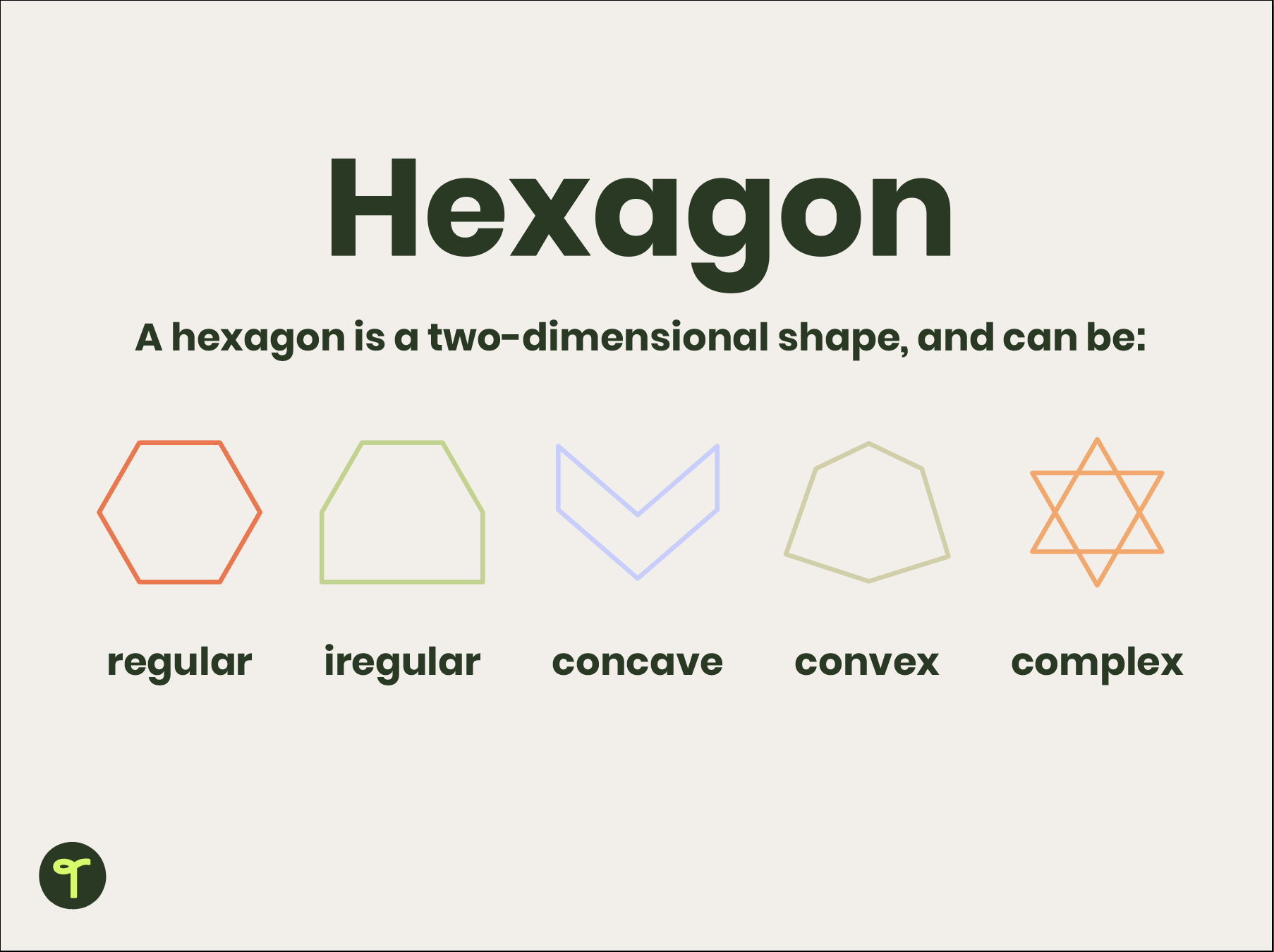# Hexagon

## What is a hexagon?

In geometry, a hexagon is a two-dimensional shape with six sides and six angles.

Hexagons are polygons. Polygons are flat two-dimensional shapes with straight sides.  Polygons enclose a space, and do not have any curved sides.

 Contents Types of hexagons What is a regular hexagon What is an irregular hexagon Symmetry of hexagons Diagonals of hexagons Are hexagons tessellating shapes? Hexagons in nature Hexagon teaching resources

## Types of Hexagons

Hexagons can be regular, irregular, concave, convex, or complex.Teaching about 2D shapes? Explore our 2D Shape teaching resource collection!

## What is a regular hexagon?

A regular hexagon is a closed-shape polygon with six equal sides and six equal angles. Every regular hexagon can be identified by specific properties.

A regular hexagon:

must be a plane figure,

must have six straight sides,

must enclose a space,

must have six interior angles measuring 120 degrees each

must have all interior angles equal to a total sum of 720 degrees.

## What is an irregular hexagon?

An irregular hexagon is a 6-sided polygon that is not regular—meaning that the sides and angles are not equal.

## Symmetry of hexagons

A regular hexagon has six rotational symmetries (rotational symmetry of order six) and six reflection symmetries (six lines of symmetry). The longest diagonals of a regular hexagon, connecting diametrically opposite vertices, are twice the length of one side of the shape.

## Diagonals of a hexagon

A diagonal is a line joining two opposite corners of a straight-sided shape.

For regular hexagons, the nine diagonals form into six equilateral triangles.

The formula to find the diagonals of hexagons is:

n (n-3)/2, where n is the number of sides of a polygon.

For a hexagon, n = 6, and 6 (6-3) / 2 equals nine diagonals.

A radius of a hexagon is the center point of the hexagon to one of its corners.

## Are hexagons tessellating shapes?

Shapes that tessellate can be repeated across a surface without leaving gaps or overlapping. Triangles and squares tessellate; circles and pentagons do not. Hexagons (which are themselves composed of tessellated triangles) do tesselate. Because hexagons are tessellating shapes, they are often used in tiling patterns and in construction.

## Hexagons in nature

The most commonly known natural hexagon is honeycomb, found in beehives. Bees are skilled at constructing uniform hexagons. Bees’ eyes are made up of many thousands of hexagonal lenses.

Snowflakes and certain crystals are also hexagonal shapes.